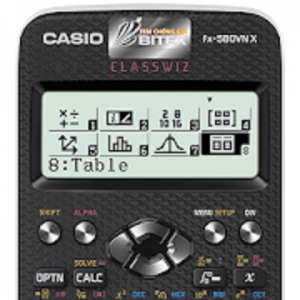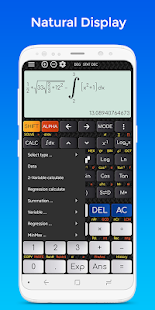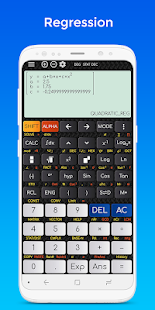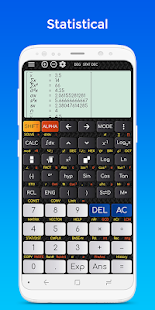# Calculator

As a user of Casio calculators, I am confident that the fx 991 EX is quite possibly the best classroom calculator they have ever produced.

Aimed squarely at the Secondary school, this would be the calculator of choice for any student.
Some of the new features that make this compelling for me are as follows:

NUMBER
The incredibly high definition screen and multi-line display options make it easy to enter calculations and see answers in textbook style and clarity – be they with fractions, brackets, surds (roots), or any combination.
The new Random Integer function makes it easy to simulate coin flips, dice rolls and even card selections or lottery draws, opening up possibilities for a huge variety of mathematical experiments and games.
Factorising large numbers into powers of prime factors with a single FACT command – amazing.

ALGEBRA
Incredible power to solve equations and systems of equations or inequalities. The machine will solve algebraically any polynomial equation up to 4th degree (quartics). It can find a root for any general equation using Newton-Raphson to refine a user-supplied initial approximation.
Similarly systems of simultaneous equations now go up to four equations in four unknowns. Also, by using the Inequality function on a quadratic, cubic or quartic polynomial, one can quickly find the points on the graph (if any) where it crosses the x-axis.

MATRICES
Matrices can now be input up to 5 x 5, with the calculator happily finding determinants, sums, products and even inverses. The previous limit was 3 x 3 matrices, although even this was sufficient to handle most exam questions.

STATISTICS
This was, for me, the main reason for my purchase. I can now finally dispense with my statistical tables – Binomial, Poisson and even Normal distributions are now covered completely. For example, one can calculate the probability that three coins out of five will show Heads (0.2734375); the probability that a person is taller than two standard deviations from the mean (0.05399096651); a two-tailed 95% Normal confidence interval (+/- 1.959964028) etc. All with a few taps – just entering the values and reading off the result.  Note to CASIO – add in Chi-Squared values and you will have all the lab scientists and social scientists queueing up as well!
– Mean, Sum, Population Standard Deviation, Sample Standard Deviation, Min, Max, Median, Q1, Q3

REGRESSION
– Linear regression
– Logarithm regression
– E exponential regression
– ab exponential regression
– Power regression
– Inverse regression

OTHER FUNCTIONS
Vectors – including Scalar and Cross Product up to 3-D vectors
Calculus – numerical differentiation and integration
Complex Numbers – Work with both Modulus/Argument and Cartesian form

SUMMARY
Natural display, powerful new functions and the same ease of use in an affordable calculator from one of the world’s most trusted calculator manufacturers make this the most highly recommended product for school use currently on the market.

What’s New
# 3.4.7
– Add math formulas to favorite
– 1000+ Math formulas
– Fixed some bugs and minor improvements

Screenshots

•••